# Nonlinear Techniques and Devices in Optical Signal Processing

Current lightwave systems perform signal processing mostly in the electric domain. This approach is acceptable if signal processing is done at the transmitter and receiver ends but becomes impractical if it needs to be carried out at intermediate nodes of an optical network. For example, switching of individual WDM channels at an intermediate node may require a change in its carrier wavelength. An electric-domain implementation would require recovering the electric bit stream with an optical receiver and then recreating the WDM channel using an optical transmitter operating at the new wavelength. An all-optical approach would simply send the channel to a nonlinear optical device (called the wavelength converter) that changes the carrier wavelength without affecting its data contents. Another example is provided by optical regenerators that clean u pan optical signal and amplify it without any optical to electrical conversion. This tutorial focuses on a variety of signal processing devices that make use of the same nonlinear effects, such as self-phase modulation (SPM), cross-phase modulation (XPM), and four-wave mixing (FWM), that are otherwise harmful for lightwave systems.

The main features of the three nonlinear phenomena, SPM, XPM, and FWM, that are useful for all-optical signal processing have been discussed in the nonlinear optical effects tutorial. All three can be implemented using a piece of optical fiber designed to enhance the nonlinear effects. Such fibers are known as highly nonlinear fibers and are designed such that the effective area of the fundamental mode is reduced considerably compared to that of standard fibers. As a result, the nonlinear parameter γ, defined to scale inversely with the effective mode area Aeff, is enhanced considerably. Its value typically exceeds 10 W-1/km for silica-based highly nonlinear fibers and becomes even larger than 1000 W-1/km for specially designed non-silica fibers.

Several devices have been developed in recent years for the purpose of optical signal processing of telecommunication signals. This section covers devices such as Sagnac interferometers, parametric amplifiers, semiconductor optical amplifiers, and bistable resonators.

#### 1. Nonlinear Optical Loop Mirrors

A nonlinear optical loop mirror (NOLM) is an example of a Sagnac interferometer in which different nonlinear phase shifts acquired by counterpropagating waves inside a fiber loop are exploited for optical signal processing. The following figure shows schematically how a Sagnac interferometer can be made by connecting a piece of long fiber to the two output ports of a fiber coupler to form a loop. The entering optical field is split into two counterpropagating parts that share the same optical path and interfere at the coupler coherently. The relative phase difference between the counterpropagating beams determines whether an input beam is reflected or transmitted by the Sagnac interferometer. In fact, if a 3-dB fiber coupler is used, any input is totally reflected, and the Sagnac loop acts as a perfect mirror. Such a device can be designed to transmit a high-power signal while reflecting it at low power levels, thus acting as an all-optical switch.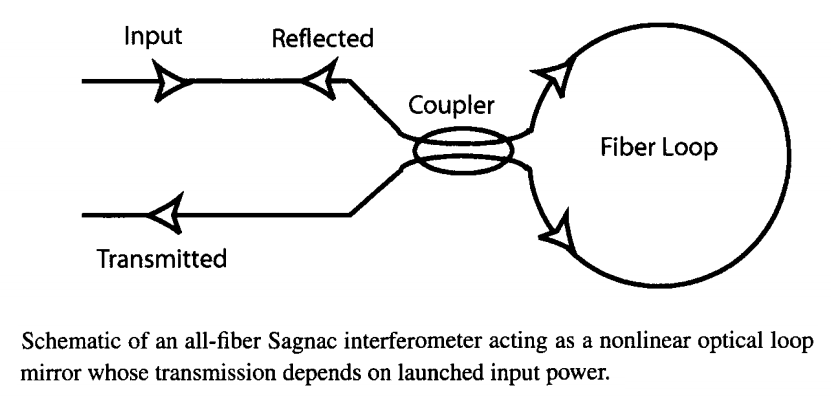The physical mechanism behind nonlinear switching can be understood by considering a CW or quasi-CW input beam. When such an optical signal is incident at one port of the fiber coupler, it's split into two parts whose relative amplitude and phases depend on the transfer matrix of the coupler given by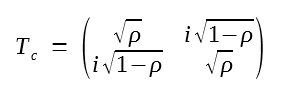where ρ represents the fraction of the input power P0 remaining in the bar port of the coupler. Using this transfer matrix, the transmittivity of a NOLM of length L is found to bewhere P0 is the input power. For ρ = 0.5, Ts equals zero, and all the input power is reflected back (hence the name optical loop mirror). Physically, if the power is equally divided between the counterpropagating waves, nonlinear phase shifts are the same for both waves, resulting in no relative phase difference between the counterpropagating waves. However, if the power-splitting factor ρ is different than 0.5, a NOLM exhibits different behavior at low and high powers and can act as an optical switch.

The following figure shows the transmitted power as a function of P0 for two values of ρ. At low powers, little light is transmitted if ρ is close to 0.5 since Ts ≈ 1 - 4ρ(1-ρ). At high powers, the SPM-induced phase shift leads to 100% transmission of the input signal whenever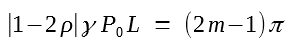where m is an integer.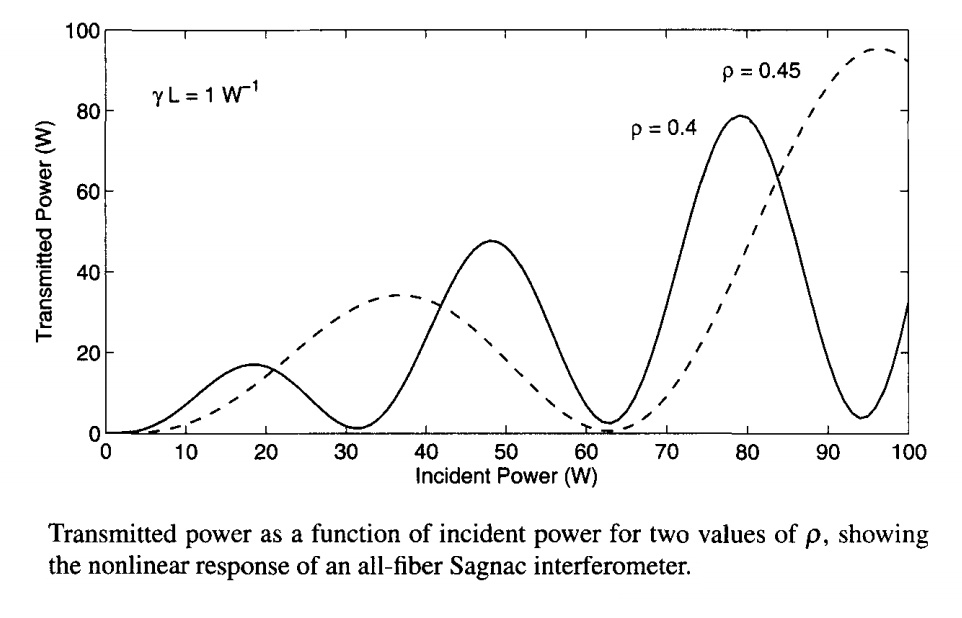As seen in the figure above, the device switches from low to high transmission periodically as the input power increases. In practice, only the first transmission peak (m = 1) is likely to be used for switching because it requires the least power. The switching power for m = 1 can be estimated from the equation above and is 31 W for a 100-m-long fiber loop when ρ = 0.45 and γ = 10 W-1/km. It can be reduced by increasing the loop length, but one should then consider the effects of fiber loss and GVD that were neglected in deriving the equation above.

The switching power of a Sagnac interferometer can also be reduced by incorporating a fiber amplifier within the loop. If the amplifier is located close to the fiber coupler, its presence introduces an asymmetry as the counterpropagating pulses are not amplified simultaneously. Since the Sagnac interferometer is unbalanced by the amplifier, even a 50:50 coupler (ρ = 0.5) can be used. The switching behavior can be understood by noting that one wave is amplified at the entrance to the loop while the counterpropagating wave experiences amplification just before exiting the loop. Since the intensities of the two waves differ by a large amount throughout the loop, the differential phase shift can be quite large. Assuming that the clockwise wave is amplified first by a factor of G, the transmittivity is found to beThe condition for complete transmission is obtained from the previous equation by replacing (1 - 2ρ) with (1 - ρ - Gρ). For ρ = 0.5, the switching power is given by (using m = 1)Since the amplification factor G can be as large as 30 dB, the switching power is reduced by a factor of up to 1000. Such a device, referred to as the nonlinear amplifying loop mirror, can switch at peak power levels below 1 mW. In a demonstration of the basic concept, 4.5 m of Nd-doped fiber was spliced within the 306-m fiber loop formed using a 3-dB coupler, and CW-like switching was observed using 10-ns pulses. The switching power was about 0.9 W even when the amplifier provided only a 6-dB gain (a factor of 4). In a later experiment, the use of a semiconductor optical amplifier, providing different gains for counterpropagating waves inside a 17-m fiber loop, resulted in switching powers of less than 250 μW when 10-ns pulses were injected into the loop.

From the standpoint of optical signal processing, XPM-induced switching is more important than SPM-induced switching because one can use another optical beam to control the switching process. In this important class of applications, a control (or a pump) beam is injected into the NOLM such that it propagates in only one direction and induces a nonlinear phase shift on one of the counterpropagating waves through XPM, while the other is not affected by it. In essence, the control signal is used to unbalance the Sagnac interferometer in a way similar to how an optical amplifier can be used to produce different SPM-induced phase shifts. As a result, the loop can be made using a 50:50 coupler so that a low-power CW beam is reflected in the absence of the control but transmitted when a control pulse is applied. Many experiments have shown the potential of XPM-induced switching.

When the signal and control wavelengths are not close to each other, we should consider the walk-off effects induced by the mismatch in their group velocities. In the absence of the GVD effects, the XPM-induced relative phase shift imposed on the signal by a control pulse is given by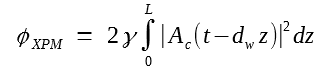where Ac is the control-pulse amplitude andrepresents the group-velocity mismatch between the control and signal pulses separated in frequency by Δω.

The integral in the equation above can be evaluated analytically for certain shapes of the control pulse. For example, for a "sech" control pulse with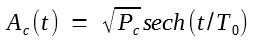the phase shift is given by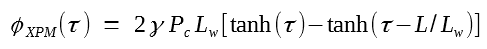where τ = t/T0 and Lw = T0/dw is the so-called walk-off length. In the case of Gaussian optical pulses with |Ac(t)|2 = Pcexp(-t2/T02), the XPM-induced phase shift becomes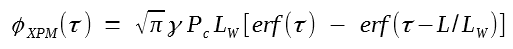where erf(x) stands for the error function.

The following figure shows the XPM-induced phase shift, normalized to its maximum value φmax = 2γPcL, as a function of τ for several values of L/LW in the case of Gaussian pulses. The temporal phase profile mimics the pulse shape for small values of L//LW, but its shape is distorted considerably as fiber length becomes larger than LW. As one may expect, the maximum value of the phase shift is reduced by the walk-off effects, an undesirable feature that increases the required peak power. However, even more detrimental from a practical standpoint is the broadening of the phase profile because it leads to a switching window wider than the control pulses.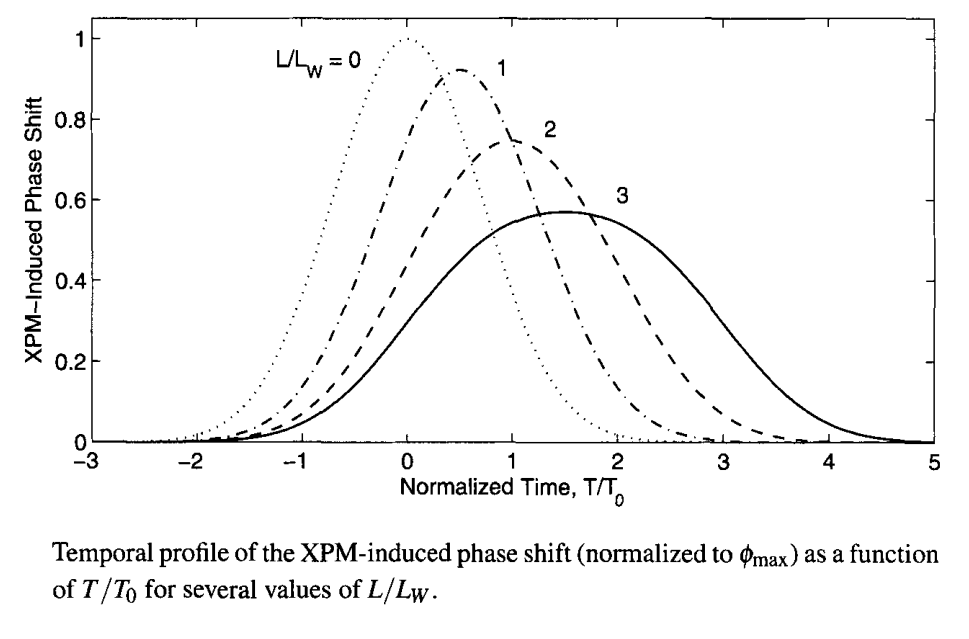The problem of pulse walk-off can be solved by using a fiber whose zero-dispersion wavelength lies between the pump and signal wavelengths such that the two waves have the same group velocity (dw = 0). Indeed, such a 200-m-long Sagnac loop was built in 1990 using polarization maintaining fiber. It was employed to switch the 1.54-μm signal using 120-ps pump pulses with 1.8-W peak power at 1.32 μm. In a later experiment, 14-ps pump pulses, obtained from a gain-switched 1.55-μm DFB laser and amplified using a fiber amplifier, were able to switch a CW signal in the wavelength region near 1.32 μm.

The pulse walk-off occurring because of the wavelength difference between the pump and signal can also be avoided by using an orthogonally polarized pump at the same wavelength as that of the signal. There is still a group-velocity mismatch because of polarization-mode dispersion but it is relatively small. Moreover, it can be used to advantage by constructing a Sagnac loop in which the slow and fast axes of polarization-maintaining fibers are interchanged in a periodic fashion. In one implementation of this idea, a 10.2-m loop consisted of 11 such sections. Two orthogonally polarized pump and signal pulses (width about 230 fs) were injected into the loop and propagated as solitons. The pump pulse was polarized along the fast axis and delayed initially such that it overtook the signal pulse in the first section. In the second section, the signal pulse traveled faster because of the reversing of slow and fast axes and overtook the pump pulse. This process repeated in each section. As a result, two solitons collided multiple times inside the Sagnac loop, and the XPM-induced phase shift was enhanced considerably.

NOLMs can be employed for many applications. The main advantage of using the nonlinearity of fibers is its ultrafast nature that permits all-optical signal processing at femtosecond time scales. The advent of highly nonlinear fibers, in which the nonlinear parameter γ is enhanced by as much as a factor of 1000, has made the use of a Sagnac interferometer much more practical by reducing the required length of the nonlinear fiber loop.

A NOLM acts as a high-pass intensity filter in the sense that it reflects low-intensity signals but transmits high-intensity radiation without affecting it. A simple application of NOLMs consists of using them for pulse shaping and pulse cleanup. For example, if a short optical pulse contains a broad low-intensity pedestal, the pedestal can be removed by passing it through such a device. Similarly, a pulse train corrupted by the amplified spontaneous emission during its amplification can be cleaned by passing it through a NOLM. The NOLM can also be used for pulse suppression and for generating a train of short optical pulses at a high repetition rate by injecting a dual-wavelength signal.

An important application of NOLMs is for converting the wavelength of a WDM channel. As early as 1992, XPM induced by control pulses from a laser operating near 1533 nm was used to convert the 1554-nm CW radiation into a pulse train. By 2000, a NOLM was used for wavelength conversion at a bit rate of 40 Gb/s. NOLMs are also useful for logic operations on digital bit streams. A polarization-maintaining Sagnac loop was used in 1991 to demonstrate elementary logic operation. NOLMs can be used as analog-to-digital and digital-to-analog converters. They are also useful for all-optical regeneration of WDM channels because they can reshape pulses while reducing the noise level. The pulse-shaping capability of such interferometers can be improved significantly by concatenating several Sagnac loops in series.

#### 2. Parametric Amplifiers

Parametric amplifiers make use of FWM inside a nonlinear medium, such as a highly nonlinear fiber, using the scheme shown schematically in the following figure.The signal at the frequency ωs that needs to be amplified is launched inside the fiber together with a CW pump of frequency ωp. The FWM phenomenon creates an idler wave at the frequency ωi = 2ωp  - ωs if the phase-matching condition is satisfied for the four photons participating in the FWM process.

Single-Pump Parametric Amplifiers

To understand the amplification process, we use the well-known theory of FWM developed for optical fibers. When a single pump is used at power levels much higher than the signal, the depletion of the pump is ignored, the FWM process is governed by two coupled linear equations written in the Fourier domain as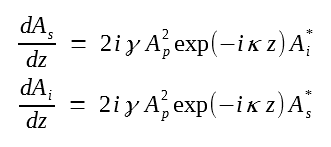where Ass, z) and Aii, z) represent the signal and idler fields, Ap is the input pump field, and κ represents the total phase mismatch given by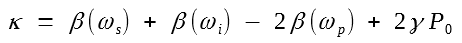where β(ω) is the propagation constant of the fiber mode at frequency ω and P0 ≡ |Ap|2 is the input pump power. It follows from the two equations above that the amplitude of the idler field is related to the complex conjugate of the signal field or, equivalently, its spectrum is inverted with respect to the signal spectrum. As was seen in the dispersion compensation with optical phase conjugation tutorial, this phase-conjugation property of FWM is useful for dispersion compensation.

The two coupled linear equations above can be solved easily to study how the signal and idler waves grow along the fiber length as a result of FWM. Using this solution, the signal and idler powers at the fiber output (z = L) are given by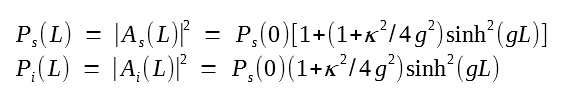where the parametric gain g is defined asThe amplification factor is obtained from the first equation above and can be written using the g equation in the form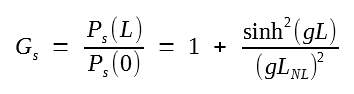where the nonlinear length is defined as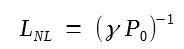The parametric gain depends on the phase mismatch κ and can become quite small if the phase-matching condition is not satisfied. On the other hand, when phase matching is perfect (κ = 0) and gL ≫ 1, the amplifier gain increases exponentially with P0 asIn terms of the nonlinear length, the gain increases as exp(2L/LNL) for a parametric amplifier as long as gain saturation and pump depletion remain negligible. Notice that the amplifier length must be longer than the nonlinear length LNL for realizing significant gain and that Gp exceeds 28 dB for L = 4LNL. Since LNL = 10 m for a fiber with γ = 100 W-1/km and pumped with P0 = 1 W, such a 50-m-long parametric amplifier can provide more than 30-dB gain.

The gain spectrum is obtained from the first Gs equation above by plotting Gp as a function of pump-signal detuning defined as δ = ωs - ωp. Assuming that the pump wavelength is located close to the zero-dispersion wavelength of the fiber, and expanding β(ω) in a Taylor series around the pump frequency ωp, we find that κ ≈ β2δ2 + 2γP0, where β2 is the GVD parameter at the pump frequency. The following figure shows the gain as a function of signal detuning from the pump wavelength at three pump-power levels for a 500-m-long parametric amplifier designed using a fiber with γ = 10 W-1/km and β2 = -0.5 ps2/km. Both the peak gain and the amplifier bandwidth increase as pump power is increased. The peak value of the gain is close to 38 dB at a 1-W pump level and occurs when the signal is detuned by 1 THz (about 8 nm) from the pump wavelength. Experimental results on parametric amplifiers agree with this simple FWM theory as long as pump depletion is negligible.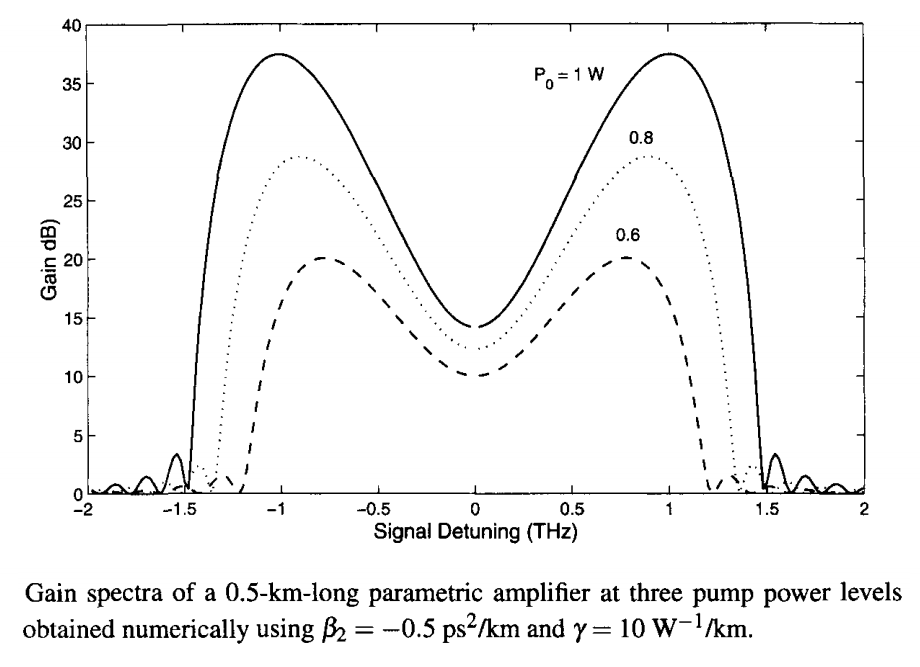Dual-Pump Parametric Amplifiers

A fundamental shortcoming of single-pump parametric amplifiers is obvious from the figure above. Because the gain is far from being uniform over the entire bandwidth, only a small portion of the gain spectrum near the two gain peaks can be used in practice. This problem can be solved by using two pumps whose wavelengths are chosen to produce a flat gain profile over a wide spectral range, while also allowing polarization-independent operation of the device.

A dual-pump parametric amplifier is fundamentally different from the conventional single-pump device. Its operating principle can be understood from the figure below where the two pumps are located on opposite sides of the zero-dispersion wavelength of the fiber.When either pump is used in isolation, the parametric gain is narrowband and relatively small. More specifically, the pump in the anomalous region produces spectral features similar to those seen in the last figure, whereas the pump in the normal region exhibits almost no gain. However, when both pumps are turned on simultaneously, the gain is not only larger but the spectral range over which gain is nearly uniform is also considerably wider. It is this central flat-gain region that turns parametric amplifiers into devices that are useful for lightwave systems.

Dual-pump parametric amplifiers provide uniform gain over a wide bandwidth by balancing three distinct processes that can produce a parametric gain in multiple spectral regions. Consider, as shown in the figure above, two pumps at frequencies ω1 and ω2, and a signal at the frequency ω1+. First, the nondegenerate FWM produces an idler at ω2- through the process ω1 + ω2 → ω1+ + ω2-. Second, the ω1 pump generates an idler at ω1- through a degenerate FWM process ω1 + ω1 → ω1+ + ω1- if its wavelength lies in the anomalous dispersion region of the fiber. Third, a Bragg-scatting process produces additional gain through the combinations denoted as

ω1 + ω1+ → ω2 + ω2- ,       ω2 + ω1+ → ω1 + ω2+

Several other weak idler waves are also created but the three dominant idlers identified in the figure above must be considered for any dual-pump parametric amplifier. The relative strength of the three processes depends on the pump powers as well as on the detuning of the two pumps from the zero-dispersion wavelength. This feature allows one to produce a desired parametric response by simply choosing the pump wavelengths and powers appropriately.

The theory of dual-pump parametric amplifiers is somewhat involved because one must consider at least six fields at the frequencies shown in the figure above simultaneously for an accurate analysis. If we neglect pump depletion, the two equations found in the single-pump case are replaced by a set of four coupled equations. It turns out that the gain spectrum depends on a larger number of parameters such as pump wavelengths, zero-dispersion wavelength, and the third- and fourth-order dispersion parameters of the fiber. In one configuration that can provide flat gain over a wide bandwidth, the two pump wavelengths are chosen almost symmetrically on the opposite sides of the zero-dispersion wavelength λ0. The following figure shows examples of the gain spectra in this case at three pump-power levels. A 500-m-long highly nonlinear fiber with γ = 10 W-1/km and λ0 = 1570 nm is assumed to be pumped using two lasers at wavelengths of 1,525 and 1,618 nm. The third- and fourth-order dispersion parameters of the fiber are β3 = 0.038 ps3/km and β4 = 1 x 10-4 ps4/km, respectively. When each pump provides 500 mW of power, a relatively large and uniform gain (38 dB) occurs over a bandwidth of more than 70 nm. The central flat region is mostly due to the nondegenerate FWM process. The other two FWM processes only affect the spectral wings and lead to oscillations seen in the figure below.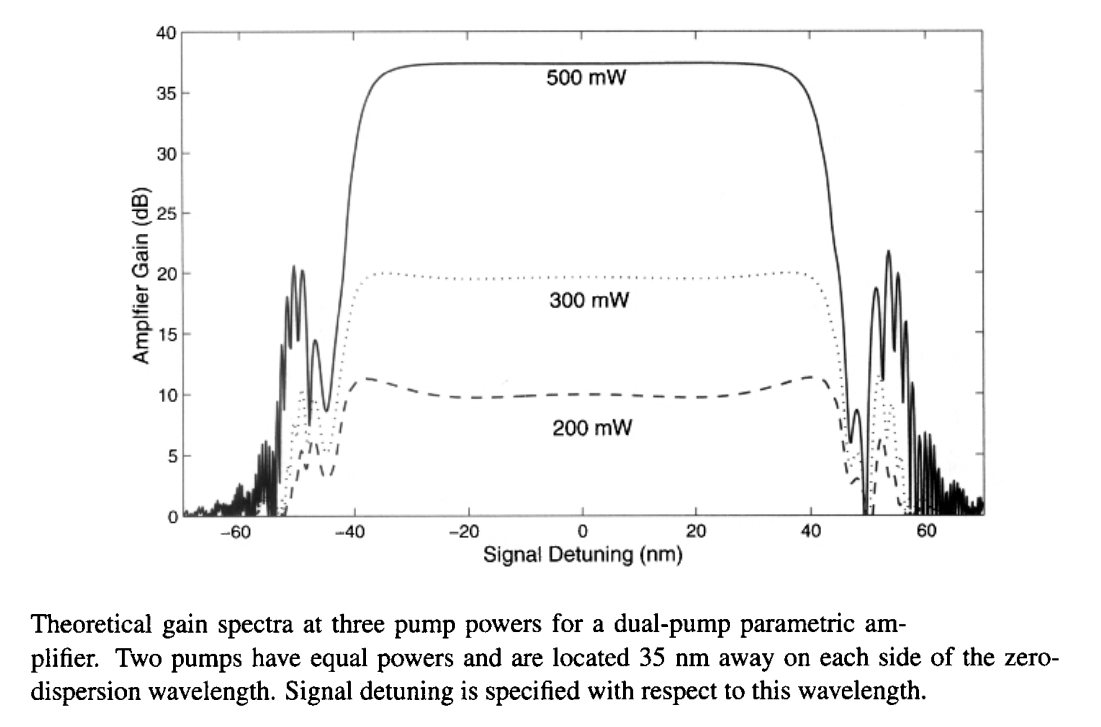Several experiments have shown that parametric amplifiers can provide flat gain over a wide bandwidth when pumped using the configuration shown in the previous figure. In one 2003 experiment, a gain of more than 40 dB was obtained over a bandwidth of 34 nm when a 1-km-long highly nonlinear fiber (Aeff = 11 μm2) with its zero-dispersion wavelength at 1,583.5 nm was pumped with 600 and 200 mW of powers at 1,559 and 1,610 nm, respectively. Unequal input pump powers were used because the Raman gain transfers power from the shorter-wavelength pump to the other pump throughout the fiber length. The onset of SBS was avoided by modulating the pump phases at 10 GHz using a pseudorandom bit pattern.

Silicon-Based Parametric Amplifiers

It is not essential to employ optical fibers for making parametric amplifiers, and any material exhibiting a large third-order susceptibility can be used in place of fibers. Recently, silicon waveguides with nanoscale dimensions (also called photonic nanowires) have been used for this purpose with considerable success.

The theory of FWM in silicon waveguides is similar to that used earlier for optical fibers with some modifications. The main change comes from the fact that when pumped in the spectral region of telecommunication interest near 1550 nm, the phenomenon of two-photon absorption (TPA) cannot be ignored because the energy of pump photons exceeds one half of the bandgap. Moreover, TPA generates free carriers that not only induce additional absorption but also change the refractive index. For this reason, the two equations should be modified to include the TPA and free-carrier effects, both of which degrade the efficiency of the FWM process. On the positive side, the nonlinear parameter γ is more than 10,000 times larger in silicon waveguides because of a much larger value of n2 for silicon and a much smaller effective mode area of silicon waveguides. As a result, FWM can be observed in short devices with lengths of only ~1 cm.

The results of FWM theory extended to include the TPA and free-carrier effects reveal that idler generation can occur over a broad bandwidth (extending > 300 nm) when pumped with a single pump whose wavelength nearly coincides with the zero-dispersion wavelength of the silicon waveguide. However, it is not possible to realize a net signal or idler amplification when the device is pumped continuously. The reason is related to the accumulation of TPA-generated free carriers whose population builds up substantially due to a relatively long lifetime of free carriers in silicon (typically > 1 ns). The figure below shows the signal gain Gs (dashed lines) and the idler conversion efficiency, ηc = Pi(L)/Ps(0) (solid curves), as a function of pump intensity for three values of the carrier lifetime τc ranging from 0.1 to 10 ns. The pump is launched at the zero-dispersion wavelength of 1551.3 nm and the signal wavelength is set at 1601.3 nm. Both Gs and ηc decrease for pump intensities > 0.2 GW/cm2 when τc exceeds 0.1 ns. This is so because more free carriers are created at high pump intensities and their density increases linearly with τc, resulting in higher losses for a larger value of τc. For τc = 10 ns, the maximum conversion efficiency is only -17.5 dB but it increases to 8 dB if τc is reduced to 1 ns.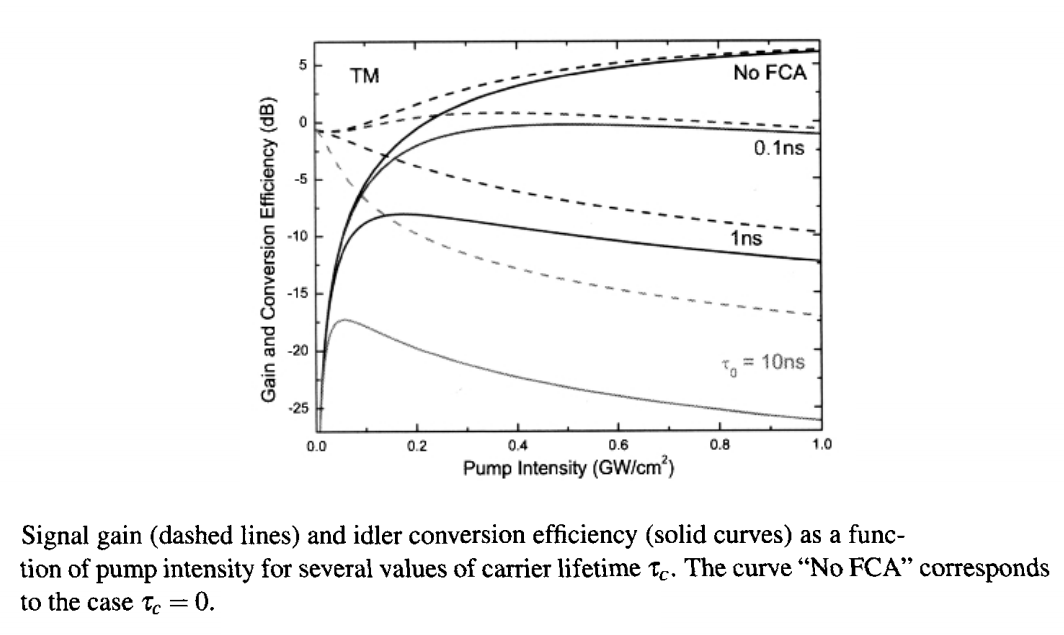The figure above indicates that, for a CW pump, signal amplification is possible only if the carrier lifetime is reduced to below 100 ps. Such a limitation does not exist for short optical pulses. For this reason, early experiments on FWM in silicon waveguides employed pulsed pumping to realize a net signal gain. However, CW pumping is often desirable for signal processing applications. The limitation imposed by the carrier lifetime can be overcome to some extent by implementing a reversed-biased p-i-n junction that removes free carriers from the FWM region by accelerating them toward the electrodes. On the positive side, a relatively short length of silicon waveguides permits FWM to occur over a broad wavelength range that can exceed 300 nm. In a 2010 experiment, a bandwidth of more than 800 nm was realized by controlling the waveguide dispersion through device dimensions. A detailed FWm theory shows that the magnitudes and signs of both the second- and fourth-order dispersions must be controlled for extending the bandwidth over which FWM can occur. In the 2010 experiment, the width and height of the silicon waveguide were controlled to realize a wavelength difference of 837 nm between the signal and idler. However, the experiment employed a CW signal and a CW pump, and the conversion efficiency was limited to below 20 dB.

#### 3. Nonlinear Effects in Semiconductor Optical Amplifiers

Semiconductor optical amplifiers (SOAs) were developed during the 1980s for their potential applications in lightwave systems. Although, with the advent of the doped-fiber and Raman amplifiers, SOAs are rarely used for fiber-loss compensation in lightwave systems, they exhibit several nonlinear effects that make them useful for optical signal processing. Because of their relatively short lengths (~1 mm), their third-order susceptibility cannot be exploited for this purpose. However, gain saturation that is intrinsic to any amplifier can be used as a substitute.

Optical Gain and its Saturation in SOAs

Although semiconductor lasers can be used as amplifiers when biased below the threshold, their bandwidth is inherently limited by multiple reflections at the facets. Most SOAs suppress this feedback using a tilted-stripe in combination with antireflection coatings at the facets. The 3-dB bandwidth can approach 100 nm for such an amplifier.

SOAs are pumped electrically by injecting electrons into its active region. If a CW optical signal is launched into its input end, this signal is amplified exponentially with the single-pass gain G = exp(gL) as long as the gain remains unsaturated. However, it is relatively easy to saturate the gain coefficient g, which is related to the electron density N by the relation

g(N) = Γσg(N - N0)

where Γ is the confinement factor, σg is the gain cross-section, and N0 is the value of N at which the SOA becomes transparent. These three parameters characterize an SOA and depend on its design details.

To discuss gain saturation, we use a well-known rate equation for the carrier density in the form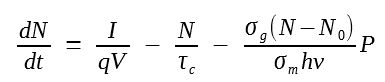where τc is the carrier lifetime and σm is the effective area of the waveguide mode. In the case of a CW beam or pulses much longer than τc, the steady-state value of N is obtained by setting dN/dt = 0. When the solution is substituted in the g(N) equation, the optical gain is found to saturate aswhere the small-signal gain g0 is given byand the saturation power Ps is defined as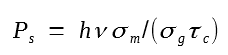Typical values of Ps are in the range of 5 to 10 mW.

SOAs as Nonlinear Devices

Gain saturation that limits the usefulness of SOAs in lightwave systems as an optical amplifier renders them quite useful for optical signal processing (while amplifying the signal simultaneously). Because of gain saturation induced by optical pulses, SOAs can be used for wavelength conversion, channel demultiplexing, and logic operations. SOAs are not only extremely compact (active volume <1 mm3), they can also be integrated monolithically with other devices on the same chip.

The most important feature of SOAs is that they exhibit a strong carrier-induced third-order nonlinearity with effective values of n2 ~ 10-13 m2/W that are seven orders of magnitude larger than that of silica fibers. Although this nonlinearity does not respond on a femtosecond time scale, it is fast enough that it can be used to make devices operating at bit rates as fast as 40 Gb/s. The origin of this nonlinearity lies in gain saturation and the fact that any change in the carrier density affects not only the optical gain but also the refractive index within the active region of an SOA.

A simple way to understand the nonlinear response of an SOA is to consider what happens when a short optical pulse is launched into it. The amplitude A(z, t) of the pulse envelop inside the SOA evolves as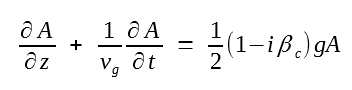where vg is the group velocity and carrier-induced index changes are included through the linewidth enhancement factor βc. The time dependence of g can be written in the form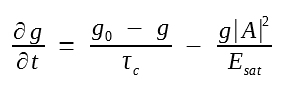where the saturation energy Esat is defined as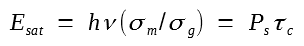Typically, Esat ~ 1 pJ.

The last three equations govern the amplification of optical pulses in SOAs. They can be solved analytically for pulses whose duration is short compared with the carrier lifetime (τp ≪ τc). The first term on the right side of the partial g equation above can then be neglected during pulse amplification. By introducing the reduced time τ = t - z/vg together with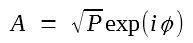The equations above can be written asThe first equation can be easily integrated over the amplifier length L to yieldwhere Pin(τ) is the input power and h(τ) is the total integrated gain defined asIf the third equation above is integrated over the amplifier length after replacing gP by ∂P/∂z, h(τ) satisfies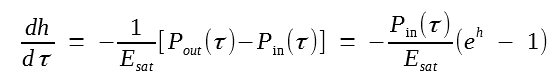This equation can easily be solved to obtain h(τ). The amplification factor G(τ) is related to h(τ) as G = exp(h) and is given by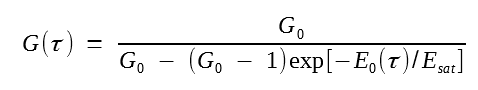where G0 is the unsaturated amplifier gain and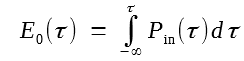is the partial energy of the input pulse defined such that E0(∞) equals the input pulse energy Ein.

The G(τ) equation above shows that the amplifier gain is different for different parts of the pulse. The leading edge experiences the full gain G0 as the amplifier is not yet saturated. The trailing edge experiences the least gain since the whole pulse has saturated the amplifier gain. Gain saturation leads to a time-dependent phase shift across the pulse. This phase shift is found by integrating the ∂φ/∂z equation above over the amplifier length and is given bySince the pulse modulates its own phase through gain saturation, this phenomenon is referred to as saturation-induced SPM. The frequency chirp is related to the phase derivative as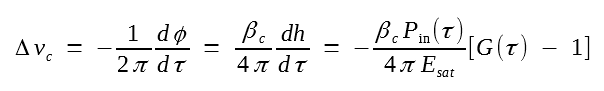SPM and the associated frequency chirp are similar to the phenomena that occur when an optical pulse propagates through a fiber. Just as in optical fibers, the spectrum of the amplified pulse broadens and contains several peaks of different amplitudes. The following figure shows the numerically calculated shape (a) and spectrum (b) of amplified pulses when a Gaussian pulse of energy such that Ein/Esat = 0.1 is amplified by a SOA. The dominant spectral peak is shifted toward the red side and is broader than the input spectrum. It is also accompanied by one or more satellite peaks. The temporal and spectral changes depend on the level of amplifier gain. The experiments performed by using picosecond pulses from mode-locked semiconductor lasers have confirmed the behavior see in this figure.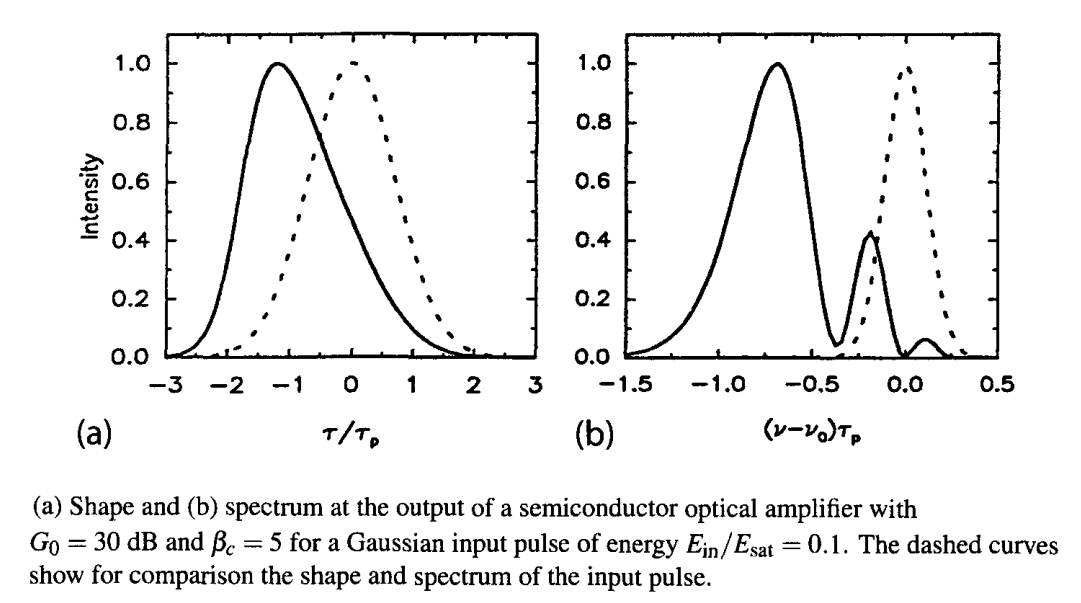SOAs also exhibit XPM when a control pulse is used to change the phase of the signal being amplified by it. Similar to the case of optical fibers, the XPM-induced phase shift can be converted into intensity changes by employing either a Mach-Zenher or a Sagnac interferometer. Such devices suffer less from the walk-off problem compared to optical fibers because their length is typically much small. When an SOA is placed within the Sagnac loop to impose the XPM-induced phase shift, the loop length can be 1 m or less because the loop is used only to propagate an input signal into the counterpropagating direction. The speed of such devices is limited inherently by the carrier lifetime (typically >0.1 ns) but it can be overcome by a clever trick. The trick consists of placing the SOA such that it is shifted from the center of the loop by a small but precise distance. It is this shift that governs the temporal window over which switching occurs rather than the carrier lifetime.

#### 4. Bistale Optical Devices

Optical bistability is a major nonlinear phenomenon that can be exploited for making bistable devices useful for optical signal processing. As the name implies, under certain conditions, the output of an optical device can have two discrete stable values for the same input. If the output can be switched between these two values through an external time-dependent control, the device acts as a time-domain switch. A simple device that exhibits optical bistability is a Fabry-Perot (FP) resonator containing a nonlinear medium. A ring resonator can also be used for the same purpose. In fact, as early as 1983, a single-mode fiber was used as the nonlinear medium inside a ring cavity to realize an optically bistable device.

The origin of optical bistability in FP resonators can be understood by using the transmissivity of an FP resonator containing a nonlinear medium. Using Rm for the mirror reflectivities, we obtain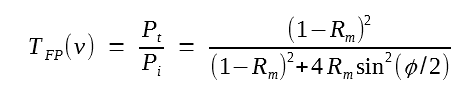where φ = δ + φNL is the total phase shift during one round trip. The linear part, δ = (Δω)τr, depends on the round-trip time τr and the frequency detuning Δω from a cavity resonance. The nonlinear contribution results from SPM and can be written as

φNL = 2γPavLm

where γ is the nonlinear parameter, Pav is the average intracavity power, and Lm is the length of nonlinear medium. For high-finesse resonators, transmitted power Pt ≈ (1 - Rm)Pav. If we use this equation in the TFP equation above, the transmitted power is found to satisfy the transcendental equation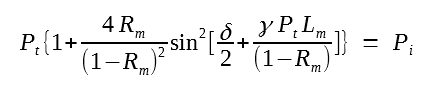It is clear from this equation that multiple values of Pt are possible at a fixed value of the incident power Pi because of the nonlinear phase shift. The number of solutions depends on the input power Pi. At low powers, only one solution exists. With increasing input power, the number of solutions increases from one to three, then to five and beyond. We focus on the case of three solutions since it requires the least input power.

Multiple solutions of the equation above lead to dispersive optical bistability, a nonlinear phenomenon that has been observed using several different nonlinear media. It occurs when the linear phase shift δ ≠ 0 so that little light is transmitted at low power levels. The nonlinear phase shift brings the signal onto an FP resonance, resulting in higher transmission. However, the transmitted power Pt does not increase linearly with Pi, as is evident from the nonlinear nature of the equation above. The following figure shows the expected behavior for three values of detuning. Over a certain range of δ, three solutions of the preceding equation produce the well-known S-shaped curve associated with optical bistability. The middle branch with a negative slope is known to be unstable. As a result, the transmitted power jumps up and down at specific values of Pi in such a way that it exhibits hysteresis. The low-output state is referred to as the "off" state, while high-output state corresponds to the "on" state. Such a device can be switched on and off by changing input power, input wavelength, or other controls that change the initial detuning δ. Indeed, any mechanism that changes the linear refractive index of the intracavity material can be used for controlling such an optical switch.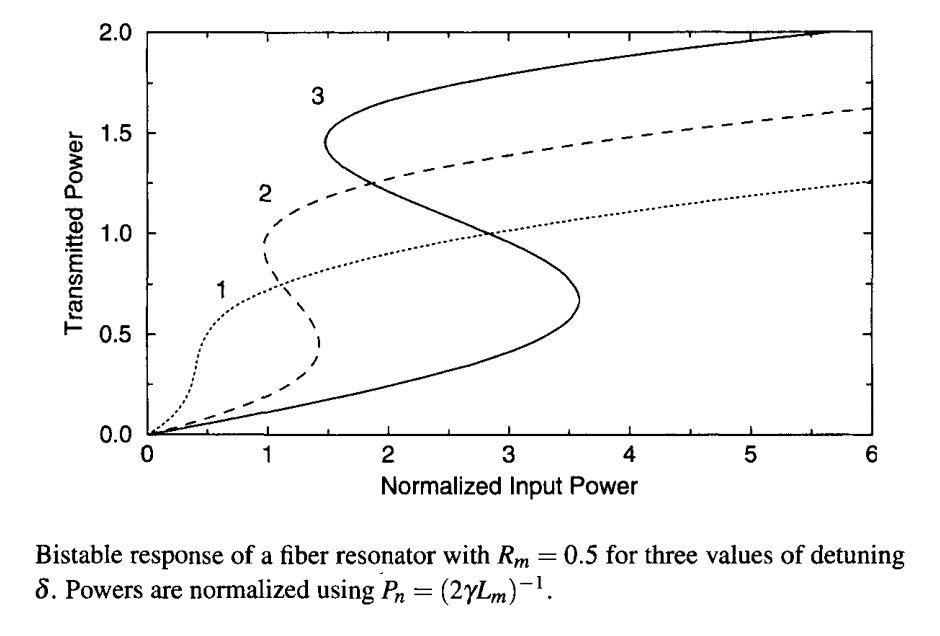Optical bistability has been observed using several different nonlinear media including semiconductor waveguides, and optical fibers. As early as 1978, a LiNbO3 waveguide was used for this purpose by coating its two cleaved ends with silver to form an FP cavity. Waveguides formed using multiple quantum wells were used during the 1980s. In the case of optical fibers, SBS hampers the observation of optical bistability when CW beams or relatively broad optical pulses are used. Bistability in a fiber-ring resonator was first seen in a 1983 experiment in which SBS was avoided using picosecond pulses. In a later experiment, SBS was suppressed by placing an optical isolator inside the ring cavity that allowed the propagation of light in a single direction. Bistable behavior was observed in this experiment at CW power levels below 10 mW. The nonlinear phase shift φNL at this power level was relatively small in magnitude (below 0.01 rad) but still large enough to induce bistability. An improved stabilization scheme was used in a 1998 experiment. Figure (a) through (d) below show the observed behavior at four values of the detuning δ. The experiment used mode-locked pulses (with a width of ~1 ps) emitted from a Ti:sapphire laser. The length of the fiber-ring resonator (about 7.4 m) was adjusted precisely so that an entering laser pulse overlapped in time with another pulse already circulating inside the cavity (synchronous pumping).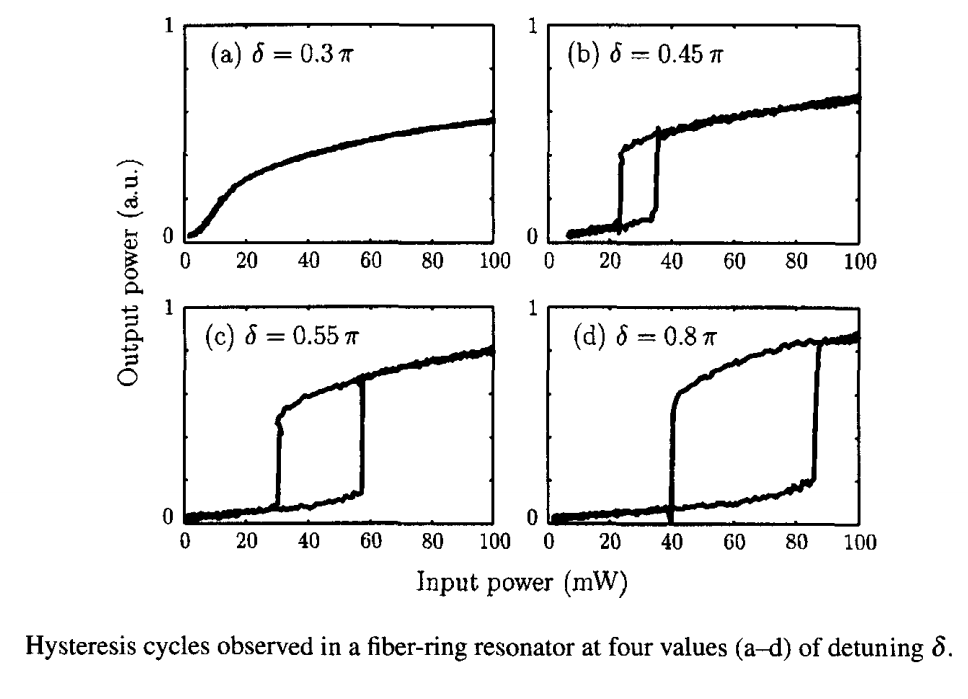The use of semiconductor lasers as optically bistable devices attracted considerable attention during the 1990s. The main advantage is that such laser employ an FP cavity by design and that the active semiconductor waveguide of the laser can provide sufficient nonlinearity for bistability to occur. Moreover, an external holding beam is not needed since lasers generate this beam internally. Thus, it is sufficient to send a control signal, provided the laser exhibits bistable behavior in some range of applied current. Most semiconductor lasers are not intrinsically bistable but can be made so by integrating one or more saturable-absorber sections within the laser cavity. Even an SOA can be used as a bistable device. Indeed, SOAs were used during the 1980s for observing bistability and for realizing all-optical flip-flops. Even though SOAs require an external holding beam, the power required is relatively low as SOAs also provide optical amplification.

FP cavities, although common, are not essential for bistability as long as there is a built-in mechanism that can provide optical feedback. Distributed feedback (DFB) from a Bragg grating formed inside a nonlinear medium can serve this purpose and lead to optical bistability. One can employ a fiber grating or a planar waveguide with the built-in grating for making time-domain switches. DFB semiconductor lasers and SOAs are natural candidates for such devices and they have been used for this purpose since the 1980s. The physical mechanism responsible for optical bistability is the dependence of the refractive index on the carrier density; as the carrier density within the active region decreases in response to gain saturation, the refractive index increases, leading to a shift of the stop band associated with the Bragg grating. This nonlinear shift of the stop band is equivalent to changing detuning the figure above. The stop band can also be shifted by changing the SOA gain through current injection. The next tutorial shows how such changes can be used for making all-optical flip-flops.